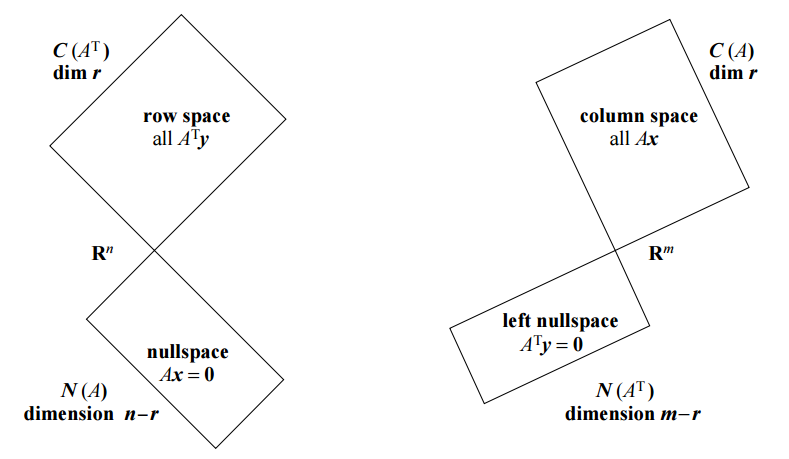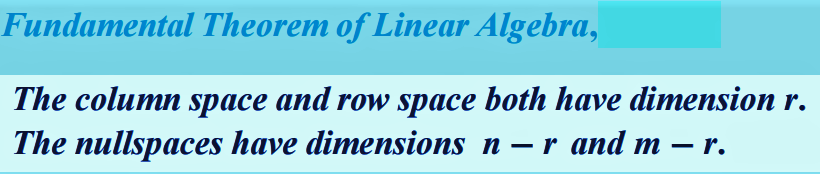### IT’S ALL ABOUT THE MATRIX:

##### MATRIX RANK:

From the UTexas:

The rank of a matrix is the number of pivots in its reduced row-echelon form (A matrix is in reduced row-echelon form if (1) it is in row echelon form, (2) all of the pivots are equal to $$1$$, and (3) all entries in the pivot columns, except for the pivots themselves, are equal to zero; matrix is said to be in row-echelon form if (1) any rows made completely of zeroes lie at the bottom of the matrix and (2) the first nonzero entries of the various rows form a staircase pattern).

Note that the rank of an $$m \times n$$ matrix cannot be bigger than $$m$$, since you can’t have more than one pivot per row. It also can’t be bigger than $$n$$, since you can’t have more than one pivot per column. If $$m < n$$, then the rank is always less than $$n$$ and there are at least $$n − m$$ columns without pivots. If $$m > n$$, then the rank is always less than $$m$$ and there are at least $$m − n$$ rows of zeroes in the reduced row-echelon form.

From Wikipedia and Caltech:

In linear algebra, the rank of a matrix $$\bf A$$ is the dimension of the vector space generated (or spanned) by its columns.

The column rank of $$\bf A$$ is the dimension (cardinality (number of vectors) of a basis of a column space) of the column space of $$\bf A$$, while the row rank of $$\bf A$$ is the dimension of the row space of $$\bf A$$.

A fundamental result in linear algebra is that the column rank and the row rank are always equal. This number (i.e., the number of linearly independent rows or columns) is simply called the rank of $$\bf A$$.

A matrix is said to have full rank if its rank equals the largest possible for a matrix of the same dimensions, which is the lesser of the number of rows and columns. A matrix is said to be rank deficient if it does not have full rank.

A matrix is full row rank when each of the rows of the matrix are linearly independent and full column rank when each of the columns of the matrix are linearly independent. For a square matrix these two concepts are equivalent and we say the matrix is full rank if all rows and columns are linearly independent. A square matrix is full rank if and only if its determinant is nonzero.

For a non-square matrix with $$m$$ rows and $$n$$ columns, it will always be the case that either the rows or columns (whichever is larger in number) are linearly dependent. Hence when we say that a non-square matrix is full rank, we mean that the row and column rank are as high as possible, given the shape of the matrix. So if there are more rows than columns $$(m>n)$$, then the matrix is full rank if the matrix is full column rank.

##### INVERTIBILITY AND NULL SPACE:

A matrix is invertible if and only if its columns are linearly independent and its rows are linearly independent. A necessary (but not sufficient) condition for this to be true is that the matrix is a square matrix.

So it is not enough for the null space to be empty, because a non-square matrix can have an empty (right) null space, as for instance:

$\begin{bmatrix}1&0\\0&1\\0&0\end{bmatrix}$

has an empty right null space, but it is not invertible. Another way of expressing the same is that the left null space also needs to be empty (in this case it is not - e.g. $$\begin{bmatrix}0&0&1\end{bmatrix}$$).

Now suppose we have a matrix $$\bf A$$ with columns $$(a_1,a_2\dots, a_n)$$ satisfying the relation $$\bf Ax=0$$ for some compatible vector $$x=(x_1,x_2,\dots,x_n)^\top$$. Then, from the rules of matrix multiplication, $$x_1a_1+x_2a_2+\dots+x_na_n=0$$, so unless $$x_1=x_2=\dots=x_n=0$$, the columns of $$\bf A$$ are not linearly independent, and hence $$\bf A$$ is not invertible. An analogous argument shows that if $$\bf y^\top A=0$$ for some compatible vector $$\bf y$$, then the rows of $$\bf A$$ are not linearly independent, so $$\bf A$$ is again not invertible.

INTUITION:

“Think about lossless data compression vs lossy data compression. In lossless compression I can recreate the original uncompressed data exactly. In lossy compression I can’t.

“Inverse transform” means a transform that recovers my original information. If my original transform didn’t throw away any information, I can invert it. If it did, I can’t.

Example:

For every two numbers $$x,y$$, record $$x+y.$$

Can I recover both $$x=3$$ and $$y=7$$ after I do the linear transformation $$x+y=10$$? No. I’ve lost information. I can’t distinguish between the transforms of $$x=3$$, $$y=7$$ and $$x=0$$, $$y=10$$. Considered as a compression algorithm that’s lossy. As a linear transform it has a $$1$$-dimensional null space. Either way, no can invert. I’ve mapped the whole line $$x=−y$$ to the origin, is the problem.

It has to be a non-empty null space to affect invertibility. The origin always maps to the origin, and I don’t lose any information that way.

If the square matrix $$\bf A$$ has a nonzero null space, $$\bf A$$ maps at least one dimension of the transformed vector space to the origin. I can’t recover that information from the transformed vector. It’s gone."

From the UTexas:

If we have a square $$n×n$$ matrix, then either the rank equals $$n$$, in which case the reduced row-echelon form is the identity matrix, or the rank is less than $$n$$, in which case there is a row of zeroes in the reduced row-echelon form, and there is at least one column without a pivot. In the first case we say the matrix is invertible, and in the second case we say the matrix is singular. The determinant of the matrix tells the difference between the two cases. The determinant of a singular matrix is always zero, while the determinant of an invertible matrix is always nonzero.

##### IF $$\bf A$$ is full column rank, then $$A^\top A$$ is always invertible:

This is the proof:

If $$A^\top Ax=0$$ for some vector $$x$$, then $$x=0:$$

$\bf 0 = x^T A^T A x = (Ax)^T(Ax) = \langle Ax, Ax \rangle = \lVert Ax \rVert^2,$

which on the other hand implies that $$\bf Ax=0$$, so since $$\bf A$$ has full rank, $$\bf x=0.$$

##### RELATIONSHIP BETWEEN DIMENSION AND RANK (Fundamental Theorem of Linear Algebra):

Key facts:

The RANK ($$r$$) of a matrix is the number of PIVOTS.

The DIMENSION of a subspace is the number of VECTORS in a BASIS.

The rank reveals the dimensions of all $$4$$ fundamental subspaces.

$\large \mathbf {A} =\begin{bmatrix} \quad & \leftarrow & n & \rightarrow \quad \\ \uparrow &\quad &\quad\\ m &\quad &\text{rank}=\color{red}{r} &\quad\\ \downarrow &\quad &\quad\\ \quad &\quad &\quad \end{bmatrix}$#### COLUMN SPACE of $$A$$ or $$C(A)$$:

1. Since it is linear combinations of columns, it is in $$\mathbb R^m$$.
2. The dimension of the column space is the rank $$\color{red}{r}.$$ There are $$r$$ vectors in a basis.
3. A basis is the pivot columns.

#### NULL SPACE of $$A$$ or $$N(A)$$:

1. Solutions of $$Ax=0$$. Hence the null space is in $$\mathbb R^n.$$
2. A basis is the special solutions. There is one for every free variable. There is an example below. So…
3. The dimension is $$n-r$$: the number of columns minus the rank (corresponding to columns with a pivot) is the number of free variables.

#### ROW SPACE $$R(A)$$: All combinations of the columns of $$A^\top$$, i.e. $$C(A^\top)$$

1. It lives in $$\mathbb R^n$$.
2. It’s dimension is $$\color{red}{r}.$$ Same as for the column space.
3. A basis for the row space is the first $$r$$ rows of the rref.

#### NULL SPACE of $$A^\top$$, i.e. $$N(A^\top)$$ - THE LEFT NULL SPACE OF A:

It’s called the left null space becasue $$A^\top \,y=0 \underset{\text{taken transpose both sides}}\implies y^T A.$$

1. It is in $$\mathbb R^m$$ because the null of $$A$$ is in $$\mathbb R^n.$$
2. The dimension is $$m-r.$$
3. We can get a basis with Gauss-Jordan $$rref\left[A_{m\times n} \quad I\right]=\left[R_{m\times n}\quad E\right]$$, so that $$EA=R.$$ We will find the basis in the $$E$$ matrix, as the rows with zero rows in $$R$$.

There is the row space and the nullspace, which together form the domain of the linear transformation: $$\mathbb R^n$$. Their intersection only contains $$1$$ element: the $$n$$ component $$0$$ vector.

There is also the column space and the left nullspace, which together form the co-domain of the linear transformation: $$\mathbb R^m$$. Their intersection only contains $$1$$ element: the $$m$$ component $$0$$ vector.

Furthermore, the rank of the matrix is the dimension of both the column space and the row space. The dimension of the nullspace is $$n−r$$, and the dimension of the left nullspace is $$m−r$$.

##### RANK-NULLITY THEOREM:

$$\text{rank(A) + null(A) = number of columns of A}$$

See this entry in MathSE for an application.

#### FREE VARIABLES AND NULL SPACE:

$\mathbf Ax=\small\begin{bmatrix}1&2&1&-3\\-2&1&-2&1\\2&0&2&-2\\1&3&1&-4\end{bmatrix}\begin{bmatrix}x_1\\x_2\\x_3\\x_4\end{bmatrix}=\begin{bmatrix}3\\-1\\2\\4\end{bmatrix}$

The reduced row echelon form of $$\mathbf A$$ is:

$\text{rref(A)}=\tiny\begin{bmatrix}1&0&1&-1\\0&1&0&-1\\0&0&0&0\\0&0&0&0\end{bmatrix}$

So you have two linearly dependent rows (only two pivot columns). Therefore the $$\text{rank(A)}=2$$, which implies that the cardinality of the column space is $$2.$$ We know that by the rank-nullity theorem that $$\text{rank(A)+ ker(A) = number of columns(A)}$$. Therefore the nullity is $$2.$$ The matrix is rank deficient, and you have two free variables.

Solving the system:

1. Find the reduced row echelon form of the augmented matrix (pivots boxed):

$\small\begin{bmatrix}1&2&1&-3&\vert&3\\-2&1&-2&1&\vert&-1\\2&0&2&-2&\vert&2\\1&3&1&-4&\vert&4\end{bmatrix}\underset{\text{Rref}}{\rightarrow}\begin{bmatrix}\boxed{1}&0&\color{blue}{1}&\color{blue}{-1}&\vert&1\\0&\boxed{1}&\color{blue}{0}&\color{blue}{-1}&\vert&1\\0&0&\color{blue}{0}&\color{blue}{0}&\vert&0\\0&0&\color{blue}{0}&\color{blue}{0}&\vert&0\end{bmatrix}$

The free variables correspond to the column vectors without a pivot row (in blue). They will be multiplied by $$x_3$$ and $$x_4$$ after solving the system, and the choice of $$x_3$$ and $$x_4$$ will make no difference, because they will be scalars in front of a vector in the null space of $$\mathbf A.$$ So we can even give them different names to identify them as free variables - for example, $$x_3=s$$ and $$x_4=t.$$ With this, we want to solve $$x_1$$ and $$x_2,$$ keeping in mind:

$x_1\begin{bmatrix}1\\0\\0\\0\end{bmatrix}+x_2\begin{bmatrix}0\\1\\0\\0\end{bmatrix}+s\begin{bmatrix}1\\0\\0\\0\end{bmatrix}+t\begin{bmatrix}-1\\-1\\0\\0\end{bmatrix}=\begin{bmatrix}1\\1\\0\\0\end{bmatrix}$

The reduced echelon spells out:

\begin{align}1\,x_1 + 0\,x_2 +1s\,-1\,t&=1;\quad x_1=1+0-1s+1t\\ 0\,x_1 + 1\,x_2 +0\,s-1t&=1; \quad x_2 =0 + 1 +0\,s+1t\\ &x_3=s\\ &x_4=t \end{align}

We end up with

$\underset{\text{vectors }\in\text{ Col(A)}}{\underbrace{\begin{bmatrix}1\\0\\0\\0\end{bmatrix}+\begin{bmatrix}0\\1\\0\\0\end{bmatrix}}}+\underset{\text{null space of A}}{\underbrace{s\begin{bmatrix}-1\\0\\1\\0\end{bmatrix}+t\begin{bmatrix}1\\1\\0\\1\end{bmatrix}}}$

$$s$$ and $$t$$ are free variables. Scalars in $$\mathbb R.$$